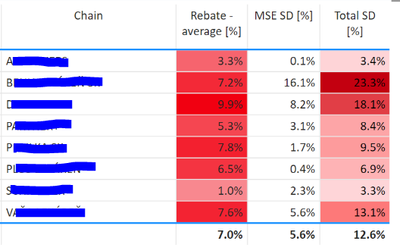cancel
Showing results for
Did you mean:Regular Visitor

## Calculating the Difference between two averages

Hello!

I would like to ask you for some advices how to calculate one new measure based on column rebate average%, MSE SD%, Total SD% seperately.

Example based on total SD% column:

1. Average for all chains is 12,6%

2. For chain "A" total SD% is 3.4%, for chain B is 23.3% and so on...

3. I would like to calculate difference between total sd% per each chain and average total sd% ---> first chain "A" --> 3.4% - total average (12.6%). PLease note that this total average changes between period.Thanks

Natalia

1 ACCEPTED SOLUTIONResolver I

Hi there,

You can use this approach (see link for file😞

``````Difference compared to average =

VAR AverageOfValue = AVERAGE('Table'[Value])

VAR AverageOfTotal =
CALCULATE(
AVERAGE('Table'[Value]),
ALLSELECTED('Table'[Chain])
)

VAR Result = AverageOfValue - AverageOfTotal

RETURN
Result``````

Using ALLSELECTED changes the average at the total row in case only a subset of chains are selected.

Regards,
Barna

8 REPLIES 8Regular Visitor

Thanks a lot. The problem has been solved.Community Support

Hi @Natalia_987 ,

Can you provide a simple PBIX file for testing? Sensitive information can be removed in advance. What kind of expected results do you expect? You can also show it with pictures. I look forward to your response.

Best Regards
Community Support Team _ Polly

If this post helps, then please consider Accept it as the solution to help the other members find it more quickly.Super User

Then there is something in your [Total SD [%]]] measure that is affecting the calculation.  Can you share a sample .pbix with dummy data so we can see the data model?

In my sample file the measure work as expected:Resolver I

Hi there,

You can use this approach (see link for file😞

``````Difference compared to average =

VAR AverageOfValue = AVERAGE('Table'[Value])

VAR AverageOfTotal =
CALCULATE(
AVERAGE('Table'[Value]),
ALLSELECTED('Table'[Chain])
)

VAR Result = AverageOfValue - AverageOfTotal

RETURN
Result``````

Using ALLSELECTED changes the average at the total row in case only a subset of chains are selected.

Regards,
BarnaSuper User

What does this measure give you?

``````Total SD % ALLSELECTED =
CALCULATE ( [Total SD [%]]], ALLSELECTED ( 'Lookup_customers (2)'[Chain] ) )``````

On every row that should give you the 12.6% total.Regular Visitor

Unfortunately, I can't see the same numbers:Regular Visitor

Hi, I believe it's not working correctly.

Please take a look at the snip attached below:Thanks

NataliaSuper User

Try a measure like this.

``````Diff =
[Total SD %] - CALCULATE ( [Total SD %], ALLSELECTED ( 'Table'[Chain] ) )``````